• 第一次玩Leetcode，对代码提交格式不了解，提交了一上午，略尴尬 leetcode确实有很多有意思的地方，不像以前做各个高校ACM练习，leetcode会给出错误信息（哪个数据的错了），也会提供测试用例自定义窗口，你可以...
第一次玩Leetcode，对代码提交格式不了解，提交了一上午，略尴尬leetcode确实有很多有意思的地方，不像以前做各个高校ACM练习，leetcode会给出错误信息（哪个数据的错了），也会提供测试用例自定义窗口，你可以自己设置测试样例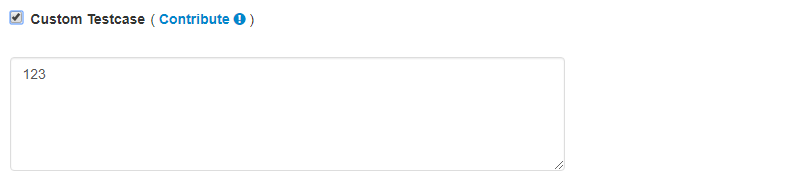对于输出结果会有个比较窗口，出现你的程序和源程序结果比对，帮你快速定位错误原因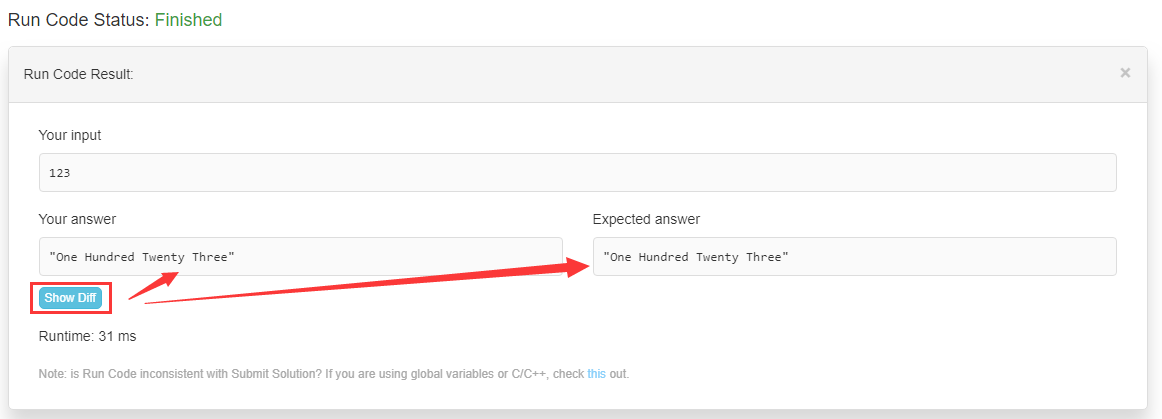此外，leetcode只需要你写核心代码部分，读取输入函数，函数调用都不需要自己做，在程序提交时，leetcode会自动补全，同时，也就要求我们写的核心代码部分必须按照leetcode规定的格式来写。

Python（像我这种习惯了C语言的人，刚开始就直接自己写了个函数就扔上面去，结果就是找不到Slution类）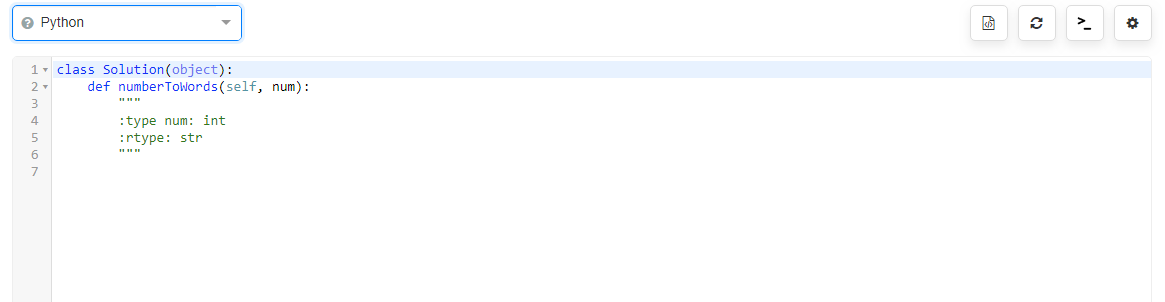C++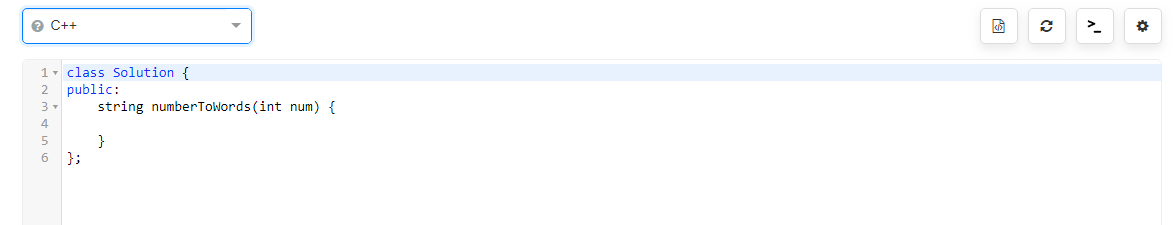JAVA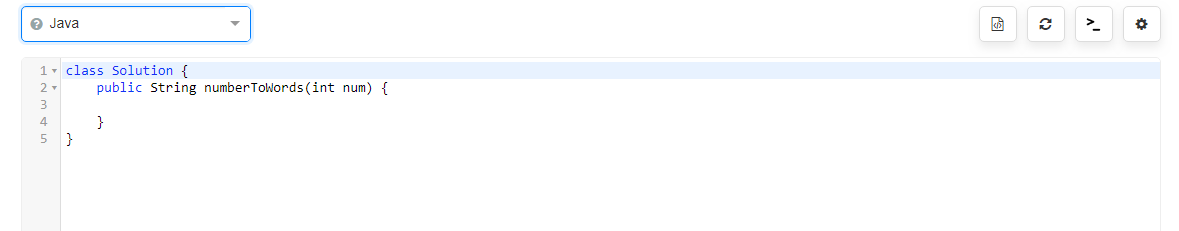C语言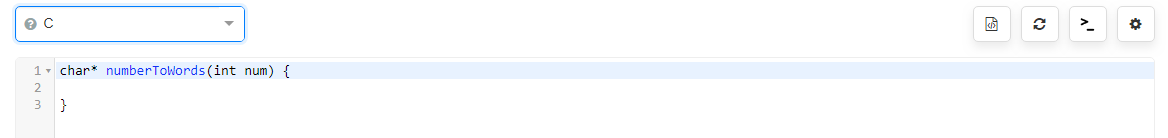主要是照着它的格式写，框框里就是leetcode提交需要的接口，你只需要实现这个接口就行了。

273. Integer to English Words

Convert a non-negative integer to its english words representation. Given input is guaranteed to be less than 231 - 1.

For example,

123 -> "One Hundred Twenty Three"
12345 -> "Twelve Thousand Three Hundred Forty Five"
1234567 -> "One Million Two Hundred Thirty Four Thousand Five Hundred Sixty Seven"

Python实现：

class Solution(object):
def __init__(self):
self.units = ['Zero', 'One', 'Two', 'Three', 'Four', 'Five', 'Six', 'Seven', 'Eight', 'Nine', 'Ten', 'Eleven',
'Twelve', 'Thirteen', 'Fourteen', 'Fifteen', 'Sixteen', 'Seventeen', 'Eighteen', 'Nineteen', 'Twenty']
self.tens = ['', 'Ten', 'Twenty', 'Thirty', 'Forty', 'Fifty', 'Sixty', 'Seventy', 'Eighty', 'Ninety']

def numberToWords(self, num):
if num > 999999999:
if num % 1000000000 == 0:
return self.numberToWords(num / 1000000000) + " Billion"
else:
return self.numberToWords(num / 1000000000) + " Billion " + self.numberToWords(num % 1000000000)
elif num > 999999:
if num % 1000000 == 0:
return self.numberToWords(num / 1000000) + " Million"
else:
return self.numberToWords(num / 1000000) + " Million " + self.numberToWords(num % 1000000)
elif num > 999:
if num % 1000 == 0:
return self.numberToWords(num / 1000) + " Thousand"
else:
return self.numberToWords(num / 1000) + " Thousand " + self.numberToWords(num % 1000)
elif num > 99:
if num % 100 == 0:
return self.units[num / 100] + " Hundred"
else:
return self.units[num / 100] + " Hundred " + self.numberToWords(num % 100)
elif num > 20:
if num % 10 == 0:
return self.tens[(num / 10)]
else:
return self.tens[(num / 10)] + " " + self.units[num % 10]
else:
return self.units[num]


展开全文• 和Luogu等其他OJ完全不一样，leetcode的代码不需要你printf，甚至不需要你有主函数。 它唯一有的就是一个叫做Solution的类，类中有一个函数，返回值和参数都给你写好了，算是一个提示吧。 在这种情况下我们提交...
博主的github链接，欢迎大家来访问~：https://github.com/Sh-Zh-7

和Luogu等其他OJ完全不一样，leetcode的代码不需要你printf，甚至不需要你有主函数。

它唯一有的就是一个叫做Solution的类，类中有一个函数，返回值和参数都给你写好了，算是一个提示吧。

在这种情况下我们提交代码就要注意以下几点：

完全不用担心头文件的问题，他都给你补全好了。
你不需要自己补全主函数，只要能够写出可以跑的目标函数即可。
如果你要引入其他函数，可以直接写在类里面。
关于leetcode还有其他几点要谈：

输入样例格式的问题：今天做到一题，对C++选手特别不友好，字符用双引号括起来，vector用Python中的list来表示。不过这个没什么影响，适应就行。
关于输入参数的问题：leetcode都把你的参数给封装好了。但是这个也有一点问题：那就是我们可能会缺少某些信息。比如他给你传一个vector，那其中就包含了vector的长度，你就应该自己去获取他，而不是以测试样例的长度作为一个常数来搞。
关于测试点数据的问题：leetcode的测试点数目很多，不单单是5个10个15个那么死板，有时候可能会有40多个；这么多测试点其中就包含了对你程序robust的考验——比如你要取绝对值，他就给你来个INT_MIN; 你不是要输入数据吗？我干脆给你一个空的。这些都很让人纠结，但是对你的代码能力也是很大的一个考验。
一些技巧：

如果你要使用类似全局变量的东西，可以在class内部声明。这样的效果就是所有的成员函数都可以使用，效果上等价于全局变量。
好了，先就讲这么多吧，以后碰到再提一下。


展开全文• LeetCode 简体中文 | English 我们的 slogon 是： 只有熟练掌握基础的数据结构与算法，才能对复杂问题迎刃有余。 :blue_book:电子书 在线阅读 这是我将我的所有公开的算法资料整理的一个电子书，全部...algorithm cpp interview
• 完成代码后，在测验详细信息视图中按s ，以将代码提交leetcode。 添加公司标签支持。 您可以将标签标记到终端代码首页视图列。 标记文件为JSON格式，可以轻松实现编辑和分享。 您可以从标签目录中找到Facebook的...
• 这篇有一些是在草稿箱里翻的，复制过来格式好像变了，间距变得很大下篇开始用新的编辑器另外发现了每次Leetcode提交答案时从detail里可以看自己的速度和别人的比较，但是好像速度和自己的机器有关？我的和第一的代码...
这篇有一些是在草稿箱里翻的，复制过来格式好像变了，间距变得很大下篇开始用新的编辑器另外发现了每次Leetcode提交答案时从detail里可以看自己的速度和别人的比较，但是好像速度和自己的机器有关？我的和第一的代码一样但就是排在了后面，这个功能相对能查看自己代码的好坏，也不用去discuss搜别人的代码了643. Maximum Average Subarray I给一个列表和一个数k，求这个列表里连续k个数的最大平均值Example 1:Input: [1,12,-5,-6,50,3], k = 4
Output: 12.75
Explanation: Maximum average is (12-5-6+50)/4 = 51/4 = 12.75我的代码：class Solution(object):
def findMaxAverage(self, nums, k):
"""
:type nums: List[int]
:type k: int
:rtype: float
"""
ans = sum(nums[:k])
sum_ = ans
for i in range(len(nums)-k):
sum_ = sum_ - nums[i]+nums[k+i]
ans = max(ans,sum_)
return ans/float(k)另一个思路：def findMaxAverage(self, A, K):
P = 
for x in A:
P.append(P[-1] + x)

ma = max(P[i+K] - P[i]
for i in xrange(len(A) - K + 1))
return ma / float(K)  大神的代码：def findMaxAverage(self, nums, k):
sums =  + list(itertools.accumulate(nums))
return max(map(operator.sub, sums[k:], sums)) / k  #迭代器中两个参数列表会在达到最短列表长度就即刻停止  #NumPy version (requires import numpy as np):
def findMaxAverage(self, nums, k):
sums = np.cumsum( + nums)
return int(max(sums[k:] - sums[:-k])) / k
20. Valid Parentheses给出由‘()[]{}’组成的字符串，判断其中的符号是否使用正确"()" and "()[]{}" are all valid but "(]" and "([)]" are not.我的代码：很耗时的reclass Solution(object):
def isValid(self, s):
"""
:type s: str
:rtype: bool
"""
last = ''
new = s
while last != new:
last,new = new,re.sub('()|()|(\{\})','',new)
return new == ''
大神的代码：思路很清晰：class Solution:
# @return a boolean
def isValid(self, s):
stack = []
dict = {"]":"[", "}":"{", ")":"("}
for char in s:
if char in dict.values():
stack.append(char)
elif char in dict.keys():
if stack == [] or dict[char] != stack.pop():
return False
else:
return False
return stack == []438. Find All Anagrams in a String给出一个两个字符串s,p求s中子字符串由p打乱组成的索引Example 1:Input:
s: "cbaebabacd" p: "abc"

Output:
[0, 6]

Explanation:
The substring with start index = 0 is "cba", which is an anagram of "abc".
The substring with start index = 6 is "bac", which is an anagram of "abc".Example 2:Input:
s: "abab" p: "ab"

Output:
[0, 1, 2]

Explanation:
The substring with start index = 0 is "ab", which is an anagram of "ab".
The substring with start index = 1 is "ba", which is an anagram of "ab".
The substring with start index = 2 is "ab", which is an anagram of "ab".我的代码：class Solution(object):
def findAnagrams(self, s, p):
"""
:type s: str
:type p: str
:rtype: List[int]
"""

pcount = dict.fromkeys('abcdefghijklmnopqrstuvwxyz',0)
pcount.update(collections.Counter(p))
scount = dict.fromkeys('abcdefghijklmnopqrstuvwxyz',0)
l = len(p)
for i in s[:l]:
scount[i] += 1
if scount == pcount:
ans = 
else:
ans = []
for j in range(len(s)-l):
scount[s[j]] -= 1
scount[s[l+j]] += 1
if scount == pcount:
ans.append(j+1)
return ans方法大同小异，有的用包含26个元素的list，或者ord对应的字典，我的代码中的字典本来只包含p中的元素，改为26个字母后减少了判断的次数，加快了算法112. Path Sum给出一个树和一个值，求是否有节点和为值的从根到叶的路径For example:Given the below binary tree and sum = 22,              5
/ \
4   8
/   / \
11  13  4
/  \      \
7    2      1
return true, as there exist a root-to-leaf path 5->4->11->2 which sum is 22.我的代码：# Definition for a binary tree node.
# class TreeNode(object):
#     def __init__(self, x):
#         self.val = x
#         self.left = None
#         self.right = None

class Solution(object):
def hasPathSum(self, root, sum):
"""
:type root: TreeNode
:type sum: int
:rtype: bool
"""
def dfs(node,sum_):
if node:
sum_ += node.val
if not(node.left or node.right):
return sum_ == sum
return dfs(node.left,sum_) or dfs(node.right,sum_)
else:
return False
return dfs(root,0)大神的代码：思路都一样，几个版本供参考# DFS Recursively
def hasPathSum1(self, root, sum):
res = []
self.dfs(root, sum, res)
return any(res)

def dfs(self, root, target, res):
if root:
if not root.left and not root.right:
if root.val == target:
res.append(True)
if root.left:
self.dfs(root.left, target-root.val, res)
if root.right:
self.dfs(root.right, target-root.val, res)

# DFS with stack
def hasPathSum2(self, root, sum):
if not root:
return False
stack = [(root, root.val)]
while stack:
curr, val = stack.pop()
if not curr.left and not curr.right:
if val == sum:
return True
if curr.right:
stack.append((curr.right, val+curr.right.val))
if curr.left:
stack.append((curr.left, val+curr.left.val))
return False

# BFS with queue
def hasPathSum(self, root, sum):
if not root:
return False
queue = [(root, sum-root.val)]
while queue:
curr, val = queue.pop(0)
if not curr.left and not curr.right:
if val == 0:
return True
if curr.left:
queue.append((curr.left, val-curr.left.val))
if curr.right:
queue.append((curr.right, val-curr.right.val))
return False下面两个是没什么头绪或者看错了题做错了半天，看了discuss的，思路非常棒205. Isomorphic Strings给两个字符串，相同的字符可以换成一个另外的相同字符，是否能通过这样的变化让两个字符串一样class Solution(object):
def isIsomorphic(self, s, t):
"""
:type s: str
:type t: str
:rtype: bool
"""
return len(set(zip(s,t))) == len(set(s)) == len(set(t))141. Linked List Cycle判断一个链表是否有循环# Definition for singly-linked list.
# class ListNode(object):
#     def __init__(self, x):
#         self.val = x
#         self.next = None

class Solution(object):
"""
:rtype: bool
"""
try:
while True:
slow = slow.next
fast = fast.next.next
if slow == fast:
return True
except:
return False                                                                                                        205. Isomorphic Strings
展开全文• 用户总提交次数6732 题目难度Easy 给你一个混合了数字和字母的字符串 s，其中的字母均为小写英文字母。 请你将该字符串重新格式化，使得任意两个相邻字符的类型都不同。也就是说，字母后面应该跟着数字，而数字后面...

重新格式化字符串 显示英文描述
通过的用户数3311
尝试过的用户数3450
用户总通过次数3455
用户总提交次数6732
题目难度Easy
给你一个混合了数字和字母的字符串 s，其中的字母均为小写英文字母。
请你将该字符串重新格式化，使得任意两个相邻字符的类型都不同。也就是说，字母后面应该跟着数字，而数字后面应该跟着字母。
请你返回 重新格式化后 的字符串；如果无法按要求重新格式化，则返回一个 空字符串 。
示例 1：
输入：s = “a0b1c2”
输出：“0a1b2c”
解释：“0a1b2c” 中任意两个相邻字符的类型都不同。 “a0b1c2”, “0a1b2c”, “0c2a1b” 也是满足题目要求的答案。
示例 2：
输入：s = “leetcode”
输出：""
解释：“leetcode” 中只有字母，所以无法满足重新格式化的条件。
示例 3：
输入：s = “1229857369”
输出：""
解释：“1229857369” 中只有数字，所以无法满足重新格式化的条件。
示例 4：
输入：s = “covid2019”
输出：“c2o0v1i9d”
示例 5：
输入：s = “ab123”
输出：“1a2b3”
提示：
1 <= s.length <= 500
s 仅由小写英文字母和/或数字组成。

这里面有几个需要考虑的，在提交的时候才发现的：
1.如果只有1个数字或者字符，应当返回本身
2.数字和字符哪个多哪个先开头
我自己写得比较复杂
class Solution:
def reformat(self, s: str) -> str:
m=[]
n=[]
result=''
if len(s)==1:
result=s
else:
for i in s:
if i in '0123456789':

m.append(i)
else:
n.append(i)

if (len(m)==len(s))|(len(n)==len(s)):
result=''
else:
if len(m)>len(n):
for i in range(len(m)+len(n)):
if i%2==0:
result+=m[int(i/2)]
else:
result+=n[int((i+1)/2-1)]
else:
for i in range(len(m)+len(n)):
if i%2==0:
result+=n[int(i/2)]
else:
result+=m[int((i+1)/2-1)]
return result

最快完成的里面 用C++和JAVA的比较多
找了一个PYTHON的
class Solution:
def reformat(self, s: str) -> str:
cc = []
nn = []
for c in s:
if c.isdigit():
nn.append(c)
else:
cc.append(c)
if abs(len(cc) - len(nn)) > 1:
return ''
if len(cc) < len(nn):
cc, nn = nn, cc
res = []
for i in range(len(cc)):
res.append(cc[i])
if i < len(nn):
res.append(nn[i])
return ''.join(res)



展开全文python 字符串
• 今天开始尝试了一下LeetCode，感觉整体格式要求比较规范，能培养规范的代码编写，而且非常注意细节，本来以为第一题非常水不需要编译直接提交也可以，用hash建立映射就可以，没想到看完答案才觉得要精益求精，力求...
• 关于LeetCode代码提交：按照LeetCode已经给出的格式写出函数，实现该接口即可。 思路：使用字典存放和查找数字与字母的映射关系；输出字符串的构成类似乘法原理（不是全排列）；可递归可for循环。 方法一：递归 ...
• 给你一个只包含 '(' 和 ')' 的字符串，找出最长有效（格式正确且连续）括号子串的长度。 示例 1： 输入：s = "(()" 输出：2 解释：最长有效括号子串是 "()" 示例 2： 输入：s = ")()())" 输出：4 解释：最长有效括号...算法 动态规划
• 但是，有一个例外：如果具有良好理论保证的算法也足够实用，可以严格胜过100％的提交，那么我将考虑实施它。 参见以获取它们的列表。 PS如果一个人不喜欢我的编码风格，他可以使用下面的代码格式化工具： ， ，或 ...
• ## leetcode1 Two Sum题解

千次阅读 2015-08-30 15:20:47
题目大概意思就是,我给你传进来一个vector... 题目刚到手的时候,发现这个与各大OJ套路不太一样啊,也就是与ACM不太一样,我还傻傻的调整输出格式什么的,而且这个是完善一个类的成员函数,而不是提交一个可以运行的完整
• 一、题目叙述：截张图算啦 二、解题思路： ...我最近在刷《算法第四版》，TwoSum的问题书中有相关...然而，第一次刷Leetcode有些许不适应，比如格式神马的还是折腾了半天，也搜了搜别人的代码，才提交成功哒！java
• 以前就写写简单的C程序，可能提交格式也是cpp，学过数据结构，但也没有接触过链表的程序，看过这道题后，真的感觉C++确实严谨。。。 题目： You are given twonon-emptylinked lists representing two non-...
• 给你一个整数 n，请你每隔三位添加点（即 “.” 符号）作为千位分隔符，并将结果以字符串格式返回。 示例 1： 输入：n = 987 输出："987" 示例 2： 输入：n = 1234 输出："1.234" 示例 3： 输入：n = 123456789 ...
• 题目内容：数字以0123456789101112131415…的格式序列化到一个字符序列中。在这个序列中，第5位（从下标0开始计数）是5，第13位是1，第19位是4，等等。请写一个函数，求任意第n位对应的数字。 上面是代码系统显示的...c++
• 无图/格式错误请到上方的文章首发页面查看。 这次代码了不得了，我提交了十次，次次0ms。 题目 给你一个数组 nums 和一个值 val，你需要 原地 移除所有数值等于 val 的元素，并返回移除后...java
• 执行用时：12 ms, 在所有C提交中击败了18.93%的用户 内存消耗：16.9 MB, 在所有C提交中击败了5.13%的用户 //本题的思路就是利用两个堆栈，当遇到左括号时，将其下标压入stack栈中，当遇到右括号时， //弹出前一...算法
• 给定一个只包含数字的字符串，复原它并返回所有可能的 IP 地址格式。 有效的 IP 地址正好由四个整数（每个整数位于 0 到 255 之间组成），整数之间用 ‘.’ 分隔。 示例: 输入: "25525511135" 输出: ["2字符串 dfs 动态规划 算法
• 解题思路： 用一个List（pathes）维护有效路径，遇到有效的路径就加进...最后将这个记录了有效路径的List（pathes）转化为String的格式并返回。 提交代码： class Solution { public String simplifyPath(String...
• 数字以0123456789101112131415…的格式序列化到一个字符序列中。在这个序列中，第5位（从下标0开始计数）是5，第13位是1，第19位是4，等等。 请写一个函数，求任意第n位对应的数字。 示例 1： 输入：n = 3 输出：3 ...
• 题目链接： ... 题目没有给出具体要求的格式，经过多次尝试提交并修正后终于AC 最终通过的程序对数字的判断符合以下几点要求： - 只考虑整数和小数的十进制情况 - 允许前导0 - 小数允许省略整数或算法
• 给定一个只包含数字的字符串，复原它并返回所有可能的 IP 地址格式。 示例: 输入: “25525511135” 输出: [“255.255.11.135”, “255.255.111.35”] 分析：这里，我们考虑递归求解。同时我们需要考虑到下面的情况...
• 给你一个字符串 time ，格式为 hh:mm（小时：分钟），其中某几位数字被隐藏（用 ? 表示）。 有效的时间为 00:00 到 23:59 之间的所有时间，包括 00:00 和 23:59 。 替换 time 中隐藏的数字，返回你可以得到的最晚...字符串 算法 java
• 首先想到的是两重for循环,提交格式啥的还出了点问题, 一定要加 throw new IllegalArgumentException("No two sum solution"); 正确源代码大概如下: class Solution { public int[] twoSum(int[] nums, int ...idea
• 添加的解决方案应该100％工作，并在leetcode提交中进行了测试。 确保添加有关问题的元数据以及时间和复杂性。 应将其作为以下格式的注释添加到文件的顶部。 /** * Question: <leetcode>. <leetcode-ques
• 1、 第一次在leetcode上刷题，写这个题，主要是为了明白leetcode上代码提交格式与其他OJ上的不同，需要根据他给出的代码，在里面完成题目的要求，不需要主函数，系统会自动调用给出的哪个函数，所有只需要写出关键的...java 算法
• 本文所有代码都在leetcode提交成功，所以输入输出的格式leetcode上题目一致，下面分析三种遍历使用到的二叉树如下图所示： 中序遍历 该二叉树的中序遍历为{4,2,5,1,6,3}。 递归实现 分析上面中序遍历后的...